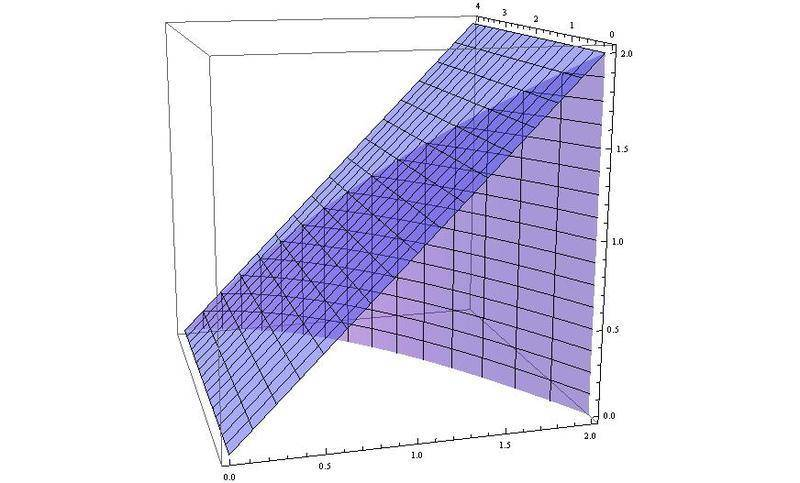# Need a hint for this triple integral

## Homework Statement

Evaluate the triple integral of sin2z/(4-z) dydzdx where the limits of integration for outer limits (x) are from 0 to 2, the middle limits (z) are 0 to 4-(x^2), and the inner limits (y) are 0 to x.

## The Attempt at a Solution

I'm not sure what the best approach is for this integral. Should I start by tackling the hardest one (dz), or work on the dx and dy integrals where the integrand is a constant? My attempt at the problem involved integrating wrt x first, then wrt y. I got the integral of 2xsin2z/(4-z) dz, from 0 to 4-x^2. I guess what's throwing me off is that trig function. Only thing I can think of is by parts...

Edit: Integrated wrt y then wrt x, got 2sin2z/4-z, so that's a bit simpler. Still need to integrate wrt z. I'm thinking by parts but wondering if there's a more efficient way that I might be missing.

Last edited:

arildno
Homework Helper
Gold Member
Dearly Missed
Hi, there!
Your major problem is that you don't have any simple antiderivative of the integrand with respect to z!

Thus, you will need to be clever, and interchange the limits of integration.

Now, to give you a tip:
$$z=4-x^{2}\to{x}=\sqrt{4-z}$$
$$0\leq{y}\leq{x}\to{0}\leq{y}\leq\sqrt{4-z}$$

Lastly, unless you have changed the integration limits, you CANNOT switch order of integration, say x-integration prior to z-integration.

Last edited:
•1 person
Hi, thank you for responding! Okay, I changed the limits of integration for y. After integrating for y and simplifying the new integrand, I got sqrt(4-z) * sin2z / 4-z = sin2z/sqrt(4-z). But since x=sqrt(4-z), then sin2z/sqrt(4-z) = sin2z/x (is that a correct statement to make?). From there, I integrate sin2z/x with respect to z from 0 to 4-(x^2). This is a complicated path to take so I just wanted to make sure I'm headed down the right track. :)

arildno
Homework Helper
Gold Member
Dearly Missed
"But since x=sqrt(4-z), then sin2z/sqrt(4-z) = sin2z/x (is that a correct statement to make?)."

Hmm..no. The UPPER LIMIT for the variable "x" equals sqrt(4-z), the variable x lies in the interval 0<=x<=sqrt(4-z)

It also seems that I made a severe messup in what I said about "y". Sorry about that!!

Thus, if we set up the entire integral, with "z" as outermost variable, we have:
$$I=\int_{0}^{4}\int_{0}^{\sqrt{4-z}}\int_{0}^{x}\frac{\sin(2z)}{4-z}dydxdz$$

•1 person
Interesting integral. Like the other two where we needed to remove the x or z in the denominator. May I also add that it might be helpful if you plotted the region. This is Mathematica code:

Code:
p1 = Plot3D[x, {x, 0, 2}, {y, 0, 4}, BoxRatios -> {1, 1, 1},
PlotStyle -> Opacity[0.5]];
p2 = ParametricPlot3D[{x, 4 - x^2, z}, {x, 0, 2}, {z, 0, 2},
RegionFunction -> Function[{x, y, z}, z <= x],
PlotStyle -> Opacity[0.5]];
Show[{p1, p2}]

Now, run the code and by interactively rotating the region as necessary, try to visualize how you can reorder the order of integration in all possible ways and then check the results numerically to verify that they agree with the way above (just a suggestion). And if you're really good, actually modify my code to include graphics which shows how the differentials are summed up like you see in the Calculus textbooks. That in my opinion helps you understand how the reordering works.#### Attachments

•1 person
@ arildno: That's all right! I appreciate all of your help and patience so far; your wonderfully detailed posts helped me see this type of integral from a different perspective, and I learned the usefulness of changing the limits. I attempted to evaluate it again, and I got (1/4)(1-cos8). (or approximately 0.286)

@ jackmell: Wow! I greatly appreciate the effort you made to represent this problem graphically. It's embarrassing, but I actually don't have/use mathematica (nor any other of the related software), but it's something I'm going to start learning pretty soon. I saved your post so that I can look back at it again in the future. In the meantime, I found this plot extremely useful in helping me visualize this region in 3-space. Thank you!